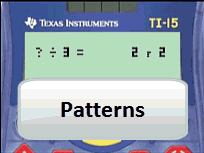# Activities

•• ##### Subject Area

• Standard: National Curriculum 7-10: Number and Algebra: Number and place value
• Standard: National Curriculum 7-10: Number and Algebra: Patterns and algebra

• ##### AuthorPrimary

90 Minutes

• ##### Device
• TI-15 Explorer™
• ##### Report an Issue

Finding Patterns#### Activity Overview

Students explore a range of number patterns using division and remainder and the reverse, multiplication and addition. The activity relates to the division algorithm and references terms such as quotient, dividend and remainder.

#### Objectives

Identify and describe factors and multiples of whole numbers and use them to solve problems - ACMNA098

Solve problems involving division by a one digit number, including those that result in a remainder - ACMNA101

#### Vocabulary

ivisor, dividend, quotient, remainder factor, composite

#### About the Lesson

Consider a number that has been divided by 3 and results in a remainder of 2. Another number is chosen, divided by 3 and again the remainder 2. The pattern continues as the next number is selected, divided by 3 and again the remainder is 2. What are the numbers and what is the pattern? Students use the unique ‘integer division’ feature of the TI-15 to display the quotient and remainder and relate this to the division algorithm. The algorithm states that each integer can be written as the product of two other integers plus the sum of a third.
Documents include Teacher notes, curriculum content, worksheets, assessment tasks and solutions.